Note: This document is for an older version of GRASS GIS that will be discontinued soon. You should upgrade, and read the current manual page.

## NAME

v.random - Generates random 2D/3D vector points.

## KEYWORDS

vector, sampling, statistics, random, point pattern, stratified random sampling, level1

## SYNOPSIS

v.random
v.random --help
v.random [-zab] output=name npoints=integer [restrict=name] [layer=string] [cats=range] [where=sql_query] [zmin=float] [zmax=float] [seed=integer] [column=name] [column_type=string] [--overwrite] [--help] [--verbose] [--quiet] [--ui]

### Flags:

-z
Create 3D output
-a
Generate n points for each individual area (requires restrict parameter)
-b
Do not build topology
Advantageous when handling a large number of points
--overwrite
Allow output files to overwrite existing files
--help
Print usage summary
--verbose
Verbose module output
--quiet
Quiet module output
--ui
Force launching GUI dialog

### Parameters:

output=name [required]
Name for output vector map
npoints=integer [required]
Number of points to be created
restrict=name
Name of input vector map
Restrict points to areas in input vector
layer=string
Layer number or name ('-1' for all layers)
A single vector map can be connected to multiple database tables. This number determines which table to use. When used with direct OGR access this is the layer name.
Default: -1
cats=range
Category values
Example: 1,3,7-9,13
where=sql_query
WHERE conditions of SQL statement without 'where' keyword
Example: income < 1000 and population >= 10000
zmin=float
Minimum z height (needs -z flag or column name)
Default: 0.0
zmax=float
Maximum z height (needs -z flag or column name)
Default: 0.0
seed=integer
The seed to initialize the random generator. If not set the process ID is used
column=name
Name of column for z values
Writes z values to column
column_type=string
Type of column for z values
Options: integer, double precision
Default: double precision

## DESCRIPTION

v.random randomly generates vector points within the current region using the selected random number generator.

v.random can generate also 3D vector points or write random value to the attribute table. Point height range or attribute value range is controlled by specifying zmin and zmax options. Both z values are included in range (zmin <= z <= zmax). Generated random attribute value type can be controlled by column_type. Use integer column type for integers and double precision for floating point numbers. Integer values are calculated by rounding random floating point number.

To produce repeatable results a random seed can be set using the option seed.

### Restriction to vector areas

If an restrict vector map with areas is specified, the location of random points is restricted to the selected areas. By default, the requested number of points are distributed across all areas.

If the -a flag is given, the requested number of points is generated for each individual area. For example, if 20 points should be generated and the input map has 100 individual areas, 2000 points will be generated in total.

Attributes attached to restrict vector map are also transferred if the layer parameter is defined > 0, see example below.

## NOTES

Importantly, attributes will only be transferred if layer > 0 (e.g., layer=1).

## EXAMPLES

All examples are based on the North Carolina sample dataset.

### Generating random points in 2D

Generate 20 random points with binary attributes (only 0 or 1):
```v.random output=binary_random npoints=20 zmin=0 zmax=1 column='binary'
v.db.select binary_random
cat|binary
1|0.63495
2|0.233421
3|0.489302
4|0.748264
5|0.505556
6|0.32975
[...]

v.univar -d binary_random
Calculating geometric distances between 20 primitives...
[...]
minimum: 148.515
maximum: 16572.8
[...]
```

### Generating random points in 2D with binary attributes

Generate 20 random points with binary attributes (only 0 or 1):
```v.random output=binary_random npoints=20 zmin=0 zmax=1 column='binary' column_type=integer
v.db.select binary_random
cat|binary
1|0
2|0
3|0
4|0
5|1
6|0
[...]
```

### Generating random points in 3D

Generate 20 random 3D points using a specific random seed:
```v.random seed=52 output=height_random npoints=40 zmin=110 zmax=170 -z
v.univar -d height_random
Calculating geometric distances between 40 primitives...
[...]
minimum: 334.889
maximum: 18351.9
range: 18017
sum: 5.38425e+06
mean: 7266.2
mean of absolute values: 7266.2
population standard deviation: 3563.95
[...]
skewness: 0.34703
```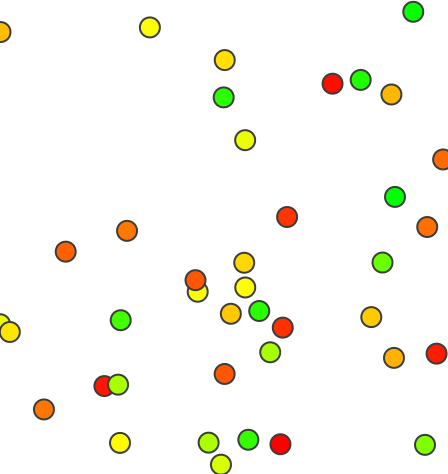Random points with different X, Y, and Z coordinates

### Generating random points in selected areas

Generate 3 random points only in selected areas ("RALEIGH" related ZIP code areas):
```v.random restrict=zipcodes_wake output=zipcodes_local_random_n3 npoints=3 where="ZIPNAME = 'RALEIGH'" -a

# visualization
d.mon wx0
d.vect zipcodes_wake
d.vect zipcodes_wake fcolor=yellow where="ZIPNAME = 'RALEIGH'"
d.vect zipcodes_local_random_n3 color=red icon=basic/circle
```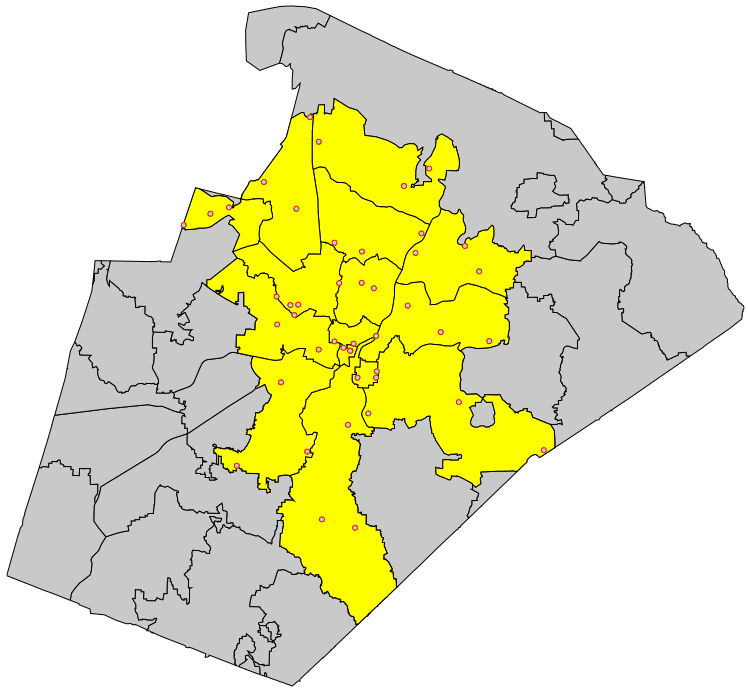Fixed number of random points generated in selected areas

To generate random adjacent areas, first the centroids are generated as points, then a triangulation is run (North Carolina sample dataset:
```g.region vector=nc_state
v.random output=randpoints6k npoints=6000
v.voronoi input=randpoints6k output=randareas6k
v.info -t randareas6k
v.category randareas6k option=print

# plot vector areas
d.mon wx0
d.vect randareas6k -c
```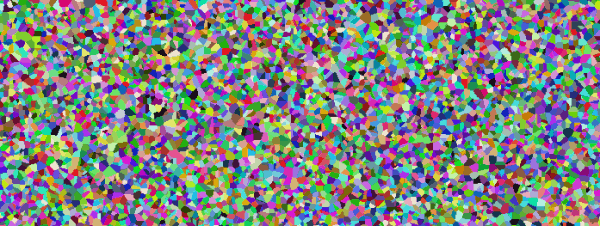Random adjacent areas from random points (here: used as centroids)

To eventually obtain isolated areas, selected areas can be extracted with v.extract.

These vector areas can also be rasterized:

```# rasterize areas
# note: rastermaps must result in at least 6k pixel in this example
g.region vector=nc_state res=500 -p -a
v.to.rast randareas6k out=randareas6k use=cat
r.colors randareas6k color=random
d.rast randareas6k
```

### Random sampling from raster map

Generate 20 random samples from a raster map:
```g.region -p raster=elevation
v.random output=random_samples npoints=20
v.db.addtable map=random_samples columns='cat INTEGER, sample DOUBLE PRECISION'
v.what.rast map=random_samples raster=elevation column=sample
v.db.select random_samples
cat|sample
1|103.9935
2|129.1266
3|96.01388
[...]
```

### Random sampling from vector map

Generate 20 random points and sample attribute data from geology (vector) map:
```g.region -p vector=geology
v.random output=random_samples npoints=20
v.db.addtable map=random_samples columns='cat integer, geology varchar(100)'
v.what.vect map=random_samples column=geology query_map=geology query_layer=1 query_column=GEO_NAME
v.db.select random_samples
cat|geology
1|PzZm
2|
3|Zatm
[...]
```

### Stratified random sampling: Random sampling from vector map by attribute

Generate 20 random points restricted to forested areas:
```g.region -p raster=landclass96
r.to.vect -v input=landclass96 output=landclass96 type=area
v.random restrict=landclass96 output=random_samples npoints=20 where="label = 'forest'" layer=1
v.db.select map=random_samples
cat|landclass96_cat|landclass96_label
1|5|forest
2|5|forest
3|5|forest
...
```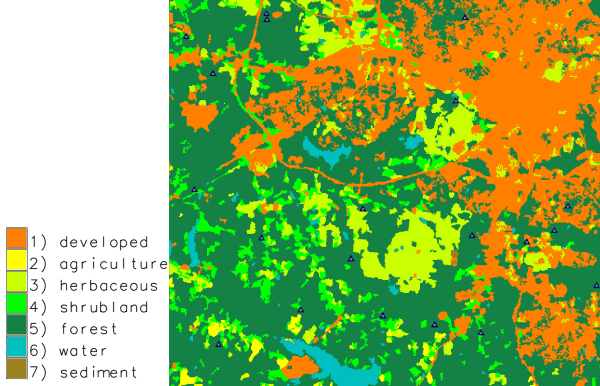Random points only sampled in forested areas (stratified random sampling)

### Stratified random sampling: Random sampling from vector map with spatial constraints

Generating n points for each individual area: in this example two random points in each water body:
```g.region -p raster=landclass96
r.to.vect -v input=landclass96 output=landclass96 type=area
v.random restrict=landclass96 output=random_samples npoints=2 where="label = 'water'" layer=1 -a
```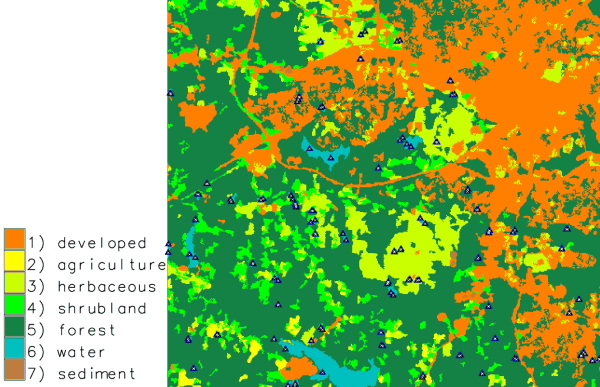Two random points sampled in each individual water body (stratified random sampling)

g.region, r.random, v.db.addtable, v.perturb, v.sample, v.univar, v.what.rast, v.what.vect

## AUTHOR

James Darrell McCauley <darrell@mccauley-usa.com>,
when he was at: Agricultural Engineering Purdue University

## SOURCE CODE

Available at: v.random source code (history)

Latest change: Monday Oct 26 11:54:36 2020 in commit: 3b6d257bdfc18a58dd42c5ab06c69ada99c56a24

Note: This document is for an older version of GRASS GIS that will be discontinued soon. You should upgrade, and read the current manual page.

© 2003-2023 GRASS Development Team, GRASS GIS 7.8.9dev Reference Manual